## k-Tuple Conjecture

The first of the Hardy-Littlewood Conjectures. The-tuple conjecture states that the asymptotic number of Prime Constellations can be computed explicitly. In particular, unless there is a trivial divisibility condition that stops,, ...,from consisting of Primes infinitely often, then such Prime Constellations will occur with an asymptotic density which is computable in terms of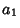, ...,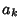. Let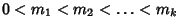, then the-tuple conjecture predicts that the number of Primessuch that,, ...,are all Prime is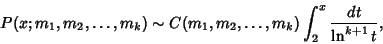(1)

where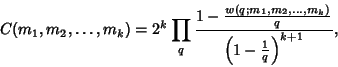(2)

the product is over Odd Primes, and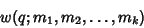(3)

denotes the number of distinct residues of 0,, ...,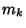(mod) (Halberstam and Richert 1974, Odlyzko). If, then this becomes(4)

This conjecture is generally believed to be true, but has not been proven (Odlyzko et al. ). The following special case of the conjecture is sometimes known as the Prime Patterns Conjecture. Letbe a Finite set of Integers. Then it is conjectured that there exist infinitely manyfor whichare all Prime Iffdoes not include all the Residues of any Prime. The Twin Prime Conjecture is a special case of the prime patterns conjecture with. This conjecture also implies that there are arbitrarily long Arithmetic Progressions of Primes.

See also Arithmetic Progression, Dirichlet's Theorem, Hardy-Littlewood Conjectures, k-Tuple Conjecture, Prime Arithmetic Progression, Prime Constellation, Prime Quadruplet, Prime Patterns Conjecture, Twin Prime Conjecture, Twin Primes

References

Brent, R. P. The Distribution of Small Gaps Between Successive Primes.'' Math. Comput. 28, 315-324, 1974.

Brent, R. P. Irregularities in the Distribution of Primes and Twin Primes.'' Math. Comput. 29, 43-56, 1975.

Halberstam, E. and Richert, H.-E. Sieve Methods. New York: Academic Press, 1974.

Hardy, G. H. and Littlewood, J. E. Some Problems of Partitio Numerorum.' III. On the Expression of a Number as a Sum of Primes.'' Acta Math. 44, 1-70, 1922.

Odlyzko, A.; Rubinstein, M.; and Wolf, M. `Jumping Champions.''

Riesel, H. Prime Numbers and Computer Methods for Factorization, 2nd ed. Boston, MA: Birkhäuser, pp. 66-68, 1994.